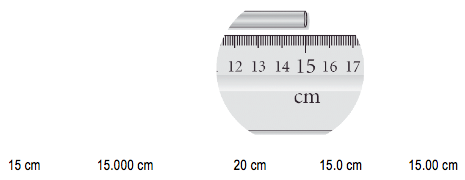Ch.1 - Intro to General ChemistryWorksheetSee all chapters
 Ch.1 - Intro to General Chemistry 2hrs & 53mins 0% complete Worksheet Ch.2 - Atoms & Elements 2hrs & 40mins 0% complete Worksheet Ch.3 - Chemical Reactions 3hrs & 25mins 0% complete Worksheet BONUS: Lab Techniques and Procedures 1hr & 38mins 0% complete Worksheet BONUS: Mathematical Operations and Functions 47mins 0% complete Worksheet Ch.4 - Chemical Quantities & Aqueous Reactions 3hrs & 30mins 0% complete Worksheet Ch.5 - Gases 3hrs & 47mins 0% complete Worksheet Ch.6 - Thermochemistry 2hrs & 28mins 0% complete Worksheet Ch.7 - Quantum Mechanics 2hrs & 35mins 0% complete Worksheet Ch.8 - Periodic Properties of the Elements 1hr & 57mins 0% complete Worksheet Ch.9 - Bonding & Molecular Structure 2hrs & 5mins 0% complete Worksheet Ch.10 - Molecular Shapes & Valence Bond Theory 1hr & 31mins 0% complete Worksheet Ch.11 - Liquids, Solids & Intermolecular Forces 3hrs & 40mins 0% complete Worksheet Ch.12 - Solutions 2hrs & 17mins 0% complete Worksheet Ch.13 - Chemical Kinetics 2hrs & 22mins 0% complete Worksheet Ch.14 - Chemical Equilibrium 2hrs & 26mins 0% complete Worksheet Ch.15 - Acid and Base Equilibrium 4hrs & 42mins 0% complete Worksheet Ch.16 - Aqueous Equilibrium 3hrs & 48mins 0% complete Worksheet Ch. 17 - Chemical Thermodynamics 1hr & 44mins 0% complete Worksheet Ch.18 - Electrochemistry 2hrs & 58mins 0% complete Worksheet Ch.19 - Nuclear Chemistry 1hr & 33mins 0% complete Worksheet Ch.20 - Organic Chemistry 3hrs 0% complete Worksheet Ch.22 - Chemistry of the Nonmetals 2hrs & 1min 0% complete Worksheet Ch.23 - Transition Metals and Coordination Compounds 1hr & 54mins 0% complete Worksheet

# Significant Figures

See all sections
Sections
Mixtures
Scientific Notation
Accuracy & Precision
Standard Deviation, Mean, Median & Mode
Metric Prefixes
Significant Figures
Energy, Heat and Temperature
Physical & Chemical Changes
Dimensional Analysis
Density
Filtration
Types of Energy
The Scientific Method
Physical & Chemical Properties

Significant Figures are used to determine some level of accuracy within our recorded measurements.

###### Significant Figures

Concept #1: Determining significant figures

Transcript

We're going to say that we know that there is some level of accuracy and precision necessary with all of our calculations. But when we get an answer, how many digits does that answer have to have? That's when significant figures come into play and play a very important role in deciding the number of digits in our answer.
Now sig figs can be really easy as long as we remember two simple rules. It has to do with decimal places or no decimal places. Let's take a look at these two rules.
We're going to say rule one has to do with if you have a decimal point. We're going to say, if your number has a decimal point, we're going to move from left to right, so we're going to move from left to right. We're going to start counting once we get to our first non-zero number and keep counting until we get to the very end.
Now, rule number two is, if our number has no decimal point. If it has no decimal point, then you're going to start moving from right to left. We're going to start counting once we get to our first non-zero number and keep counting until you get to the very end.
Now, that we've seen these two simple rules, let's apply them to these examples.

Example #1: How many sig figs does each number contain?

0.0000185 m                      749 mol

17.3 x 103 mL                       100. min

0.0010050 kg                                   1560 mol

Example #2: Read the length of the metal bar to the correct number of significant figures.###### Significant Figure Calculations

Concept #2: Understanding calculations with Significant Figures

Transcript

We’re going to say, when it comes to calculations we separate them into two categories. Multiplication and division go together; addition and subtraction will go together.
So we are going to say here when it comes to multiplication and division, measurements with the least number of significant figures, I’m just going to say sig figs, will determine our final answer. And when it comes to addition and subtraction we are going to say measurements with the least number of decimal places will determine our final answer.
So just remember when we are multiplication or division, we’re looking at the number of sig figs for our measurements. The one with the smallest number of sig figs will determine the number of sig figs in our final answer.
When we are doing addition and subtraction we want to get the fewest number of decimal positions that will give us our final answer.

The number of significant figures involved in a calculation depends on whether we are adding, subtracting, multiplying or dividing.

Example #3: Perform the following calculation to the right number of sig figs:

(3.16) x (0.003027) x (5.7 x 10-3)

Whenever we are adding or subtracting numbers with different exponents we must manipulate them to one common exponent value. We manipulate the values to get the least number of decimal places.

Example #4: Perform the following calculation to the right number of sig figs:

2.628 x 106

6.281 x 104

+ 0.827 x 107

Whenever we are dealing with a mixture of functions just remember your order of operations.

Example #5: Perform the following calculation to the right number of sig figs:

(42.00 – 40.915) • (25.739 – 25.729)

(11.50 • 1.001) +  (0.00710 • 700.)